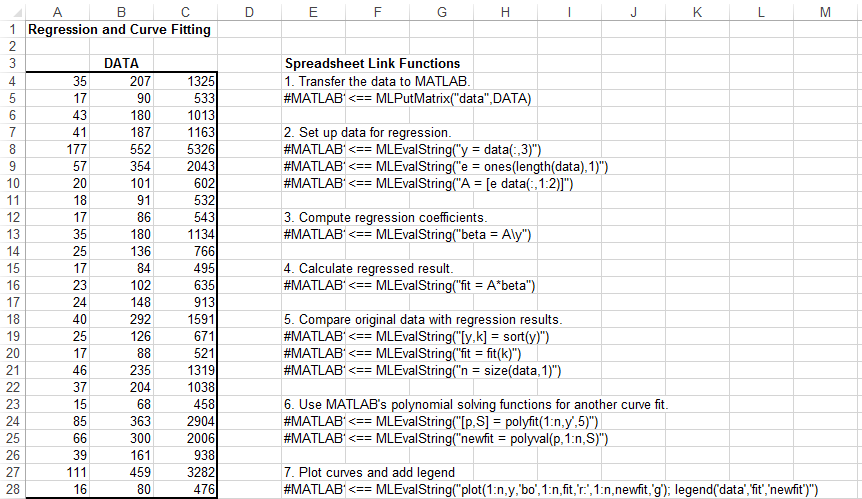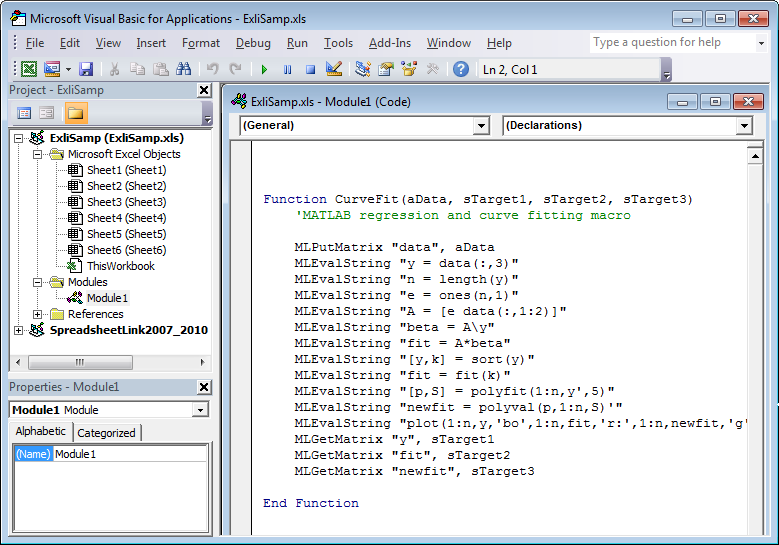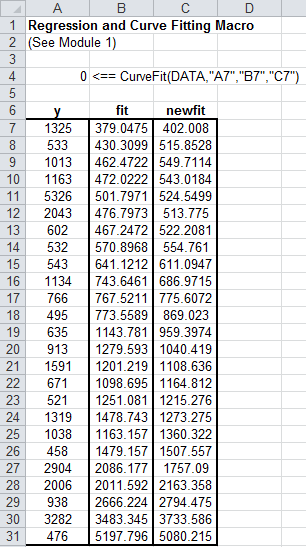## Model Data Using Regression and Curve Fitting

This example shows how to execute MATLAB® data regression and curve fitting in Microsoft® Excel® using a worksheet and a VBA macro.

The example organizes and displays the input and output data in a Microsoft Excel worksheet. Spreadsheet Link™ functions copy the data to the MATLAB workspace and execute MATLAB computational and graphic functions. The VBA macro also returns output data to a worksheet.

To work with VBA code in Excel with Spreadsheet Link, you must enable Spreadsheet Link as a reference in the Microsoft Visual Basic® Editor. For details, see Installation.

Open the `ExliSamp.xls` file and select the Sheet1 worksheet. For help finding the `ExliSamp.xls` file, see Installation.

Sheet1 of the spreadsheet contains the named range `DATA`, which consists of the example data set in worksheet cells `A4` through `C28`.### Model Data in Worksheet

To perform regression and curve fitting, execute the specified Spreadsheet Link functions in worksheet cells.

1. Execute the Spreadsheet Link function that copies the sample data set to the MATLAB workspace by double-clicking the cell `E5` and pressing Enter. The data set contains 25 observations of three variables. There is a strong linear dependence among the observations. In fact, they are close to being scalar multiples of each other.

2. Execute the functions in cells `E8`, `E9`, and `E10`. The Spreadsheet Link functions in these cells regress the third column of data on the other two columns, and create:

• A single vector `y` containing the third-column data

• A three-column matrix `A`, which consists of a column of 1s followed by the rest of the data

3. Execute the function in cell `E13`. This function calculates the regression coefficients by using the MATLAB back slash `(\)` operation to solve the overdetermined system of linear equations, `A*beta = y`.

4. Execute the function in cell `E16`. MATLAB matrix-vector multiplication produces the regressed result, `fit`.

5. Execute the functions in cells `E19`, `E20`, and `E21`. These functions:

1. Compare the original data with `fit`.

2. Sort the data in increasing order and apply the same permutation to `fit`.

3. Create a scalar for the number of observations.

6. Execute the functions in cells `E24` and `E25`. Fit a polynomial equation to the data for a fifth-degree polynomial. The MATLAB `polyfit` function automates setting up a system of simultaneous linear equations and solutions for the coefficients. The `polyval` function then evaluates the resulting polynomial at each data point to check the goodness of the fit `newfit`.

7. Execute the function in cell `E28`. The MATLAB `plot` function graphs the original data (blue circles), the regressed result `fit` (dashed red line), and the polynomial result (solid green line).Since the data is closely correlated, but not exactly linearly dependent, the `fit` curve (dashed line) shows a close, but not exact, fit. The fifth-degree polynomial curve `newfit` is a more accurate mathematical model for the data.

### Model Data Using VBA Macro

To model the data using a VBA macro, execute the Spreadsheet Link functions in a VBA macro.

1. In the `ExliSamp.xls` file, click the Sheet2 tab. The worksheet for this example appears.Cell `A4` calls the macro `CurveFit`, which you can examine in the Microsoft Visual Basic environment.While this module is open, ensure that the Spreadsheet Link add-in is enabled. To enable it, see Add-In Setup. After the add-in is enabled, the Project Explorer lists it under the References folder.

2. Execute the `CurveFit` macro by double-clicking the cell `A4` and pressing Enter. The macro runs the Spreadsheet Link functions. When the macro finishes, the input and output data appears in worksheet cells `A7:C31`.

• Column A contains the original data `y` (sorted).

• Column B contains the corresponding regressed data `fit`.

• Column C contains the polynomial data `newfit`.